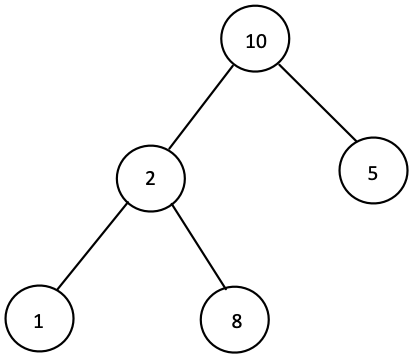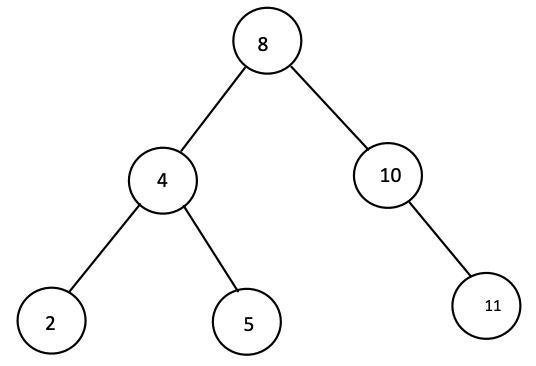# Count the Number of Binary Search Trees present in a Binary Tree

## Introduction

Let us first understand the problem statement. The problem statement goes like this: You are given a binary tree and you need to calculate the number of binary search tree present in it.

Example1:

Input:Output: 4

Explanation: There are 3 leaf nodes and 1 subtree rooted at 2 which are valid BSTs, hence our answer is 4.

Example2:

Input:Output: 6

Explanation: Every subtree in the given tree is a valid BST hence our answer is 6.

## Algorithm

Before diving into the algorithm, let us first understand what is a binary search tree.

A BST is a binary tree with the following properties:

1. The value of the root node is greater than the maximum value in the left subtree.
2. The value of the root node is smaller than the minimum value in the right subtree.

The above two conditions must hold for every possible subtree. A tree with 0 or 1 nodes is considered to be a BST.

Intuition: if a tree is a binary search tree then all of its subtrees will be binary search trees and vice versa.

## Solution Approach

• We will be following a bottom-up approach. Every node will return a chunk of data that contains four pieces of information.
• MINVAL’: stores the minimum value in the subtree.
• MAXVAL’: stores the maximum value in the subtree
• isBST’: a boolean value (true/false) that determines whether the tree is a BST or not.
• numBSTs’: stores the total number of valid BSTs in the tree.
• We will then use this data to build up our solution recursively. Every node will make two calls, one to its left child and one to its right child (if at all they are present). Each call will return a chunk of data as discussed above.
• We will then use this information to check the following condition: the left and right subtree are BST and the node’s value is greater than ‘MAXVAL’ in the left subtree and the node’s value is smaller than ‘MINVAL’ in the right subtree.
• If the above condition holds, then, this means the subtree which is currently being processed is a valid BST, else it is not. Accordingly, we will update the variables, {‘MINVAL’, ‘MAXVAL’, ‘isBST’, ‘numBSTs’} and return this chunk of data to the caller.

Let us implement the above approach.

## C++ Code Implementation

``````#include <bits/stdc++.h>
using namespace std;

// defining TreeNode's structure
struct TreeNode
{
int val;
TreeNode *left, *right;
TreeNode(int x)
{
this->val = x;
this->left = NULL;
this->right = NULL;
}
};

// defining Data's structure
struct Data
{
int maxval, minval, numBSTs;
bool isBST;
Data()
{
this->isBST = true;
this->numBSTs = 0;
this->minval = INT_MAX;
this->maxval = INT_MIN;
}
};

Data countBSTs(TreeNode *root)
{
// creating Data to be returned
Data dataRoot = Data();

// base case
if (root == NULL)
{
return dataRoot;
}

Data dataLeft = countBSTs(root->left);
Data dataRight = countBSTs(root->right);

// checking if the subtree is a BST or not
if (dataLeft.isBST and dataRight.isBST and (root->val > dataLeft.maxval and root->val < dataRight.minval))
{
dataRoot.numBSTs = dataLeft.numBSTs + dataRight.numBSTs + 1;
dataRoot.minval = min(root->val, dataLeft.minval);
dataRoot.maxval = max(root->val, dataRight.maxval);
}
else
{
dataRoot.isBST = false;
dataRoot.numBSTs = dataLeft.numBSTs + dataRight.numBSTs;
dataRoot.minval = min(root->val, min(dataLeft.minval, dataRight.minval));
dataRoot.maxval = max(root->val, max(dataLeft.maxval, dataRight.maxval));
}
return dataRoot;
}

int main()
{
TreeNode *root = new TreeNode(8);
root->left = new TreeNode(4);
root->left->left = new TreeNode(2);
root->left->right = new TreeNode(5);
root->right = new TreeNode(10);
root->right->right = new TreeNode(11);
cout << "The number of BST in the given tree is: " << countBSTs(root).numBSTs << endl;
}``````

Output:

``The number of BST in the given tree is: 6``

## Complexity Analysis

Time Complexity: The time complexity of the above approach is O(N) where ‘N’ is the number of nodes present in the given binary tree. This is due to the fact that we are traversing through all the nodes of the tree once.

Space complexity: The space complexity is O(1). This is due to the fact that we aren’t using any auxiliary space. (Note: we are not considering the recursion call stack in the space complexity analysis).

## FAQs

1. What is a binary search tree?

ANS: A binary search tree is a binary tree with the following conditions:

a. the root’s value must be greater than the maximum value in the left subtree

b. the root’s value must be smaller than the minimum value in the right subtree

c. the left and the right subtree must also be a valid BST.

2. What is the most optimised solution to count the number of BSTs in a tree?

ANS: We need to traverse through each and every node in the tree to find out whether that subtree is a BST or not. Hence, the time and space complexity of the most optimized solution is O(N) and O(1) respectively.

## Key Takeaways

In this blog, we devised a method to count the number of BSTs in a tree efficiently.

1. First, we discussed what is a binary search tree and then formulated a recursive approach to calculate the number of BSTs.
2. We did the time and space complexity analysis of the solution approach.